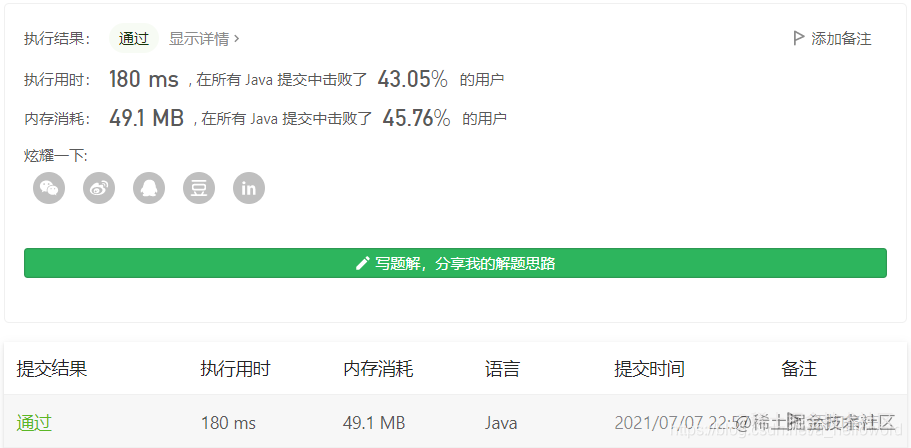# 1711. 大餐计数

#### 2021-07-07 LeetCode每日一题

``````输入：deliciousness = [1,3,5,7,9]

``````输入：deliciousness = [1,1,1,3,3,3,7]

• 1 <= deliciousness.length <= 10 ^ 5
• 0 <= deliciousness[i] <= 2 ^ 20

``````class Solution {
public int countPairs(int[] deliciousness) {
Arrays.sort(deliciousness);
int len = deliciousness.length, res = 0;
int[] bits = new int;
for (int i = 0; i <= 21; i++) {
bits[i] = (int)Math.pow(2, i);
}

for (int val : bits) {
int left = 0, right = len - 1;
while (left < right) {
int sum = deliciousness[left] + deliciousness[right];

if (sum == val) {
int tempLeft = left, tempRight = right, count = 0;
// 处理重复数字
while (tempLeft < tempRight && deliciousness[tempLeft] == deliciousness[left]) {
while (tempRight > tempLeft && deliciousness[tempRight] == deliciousness[right]) {
res = (res + 1) % (1000_000_007);
tempRight--;
}
count = tempRight;
tempRight = right;
tempLeft++;
}

left = tempLeft;
right = count;
} else if (sum < val) {
left++;
} else if (sum > val) {
right--;
}
}
}

return res;
}
}``````class Solution {
public int countPairs(int[] deliciousness) {
HashMap<Integer, Integer> map = new HashMap<>();
int ans = 0;
for(int i = 0; i < deliciousness.length; i++){
for(int j = 1; j <= 1 << 21; j <<= 1){
if(map.containsKey(j - deliciousness[i])){
ans = (ans + map.get(j - deliciousness[i])) % 1000000007;
}
}
map.put(deliciousness[i], map.getOrDefault(deliciousness[i], 0) + 1);
}
return ans;
}
}